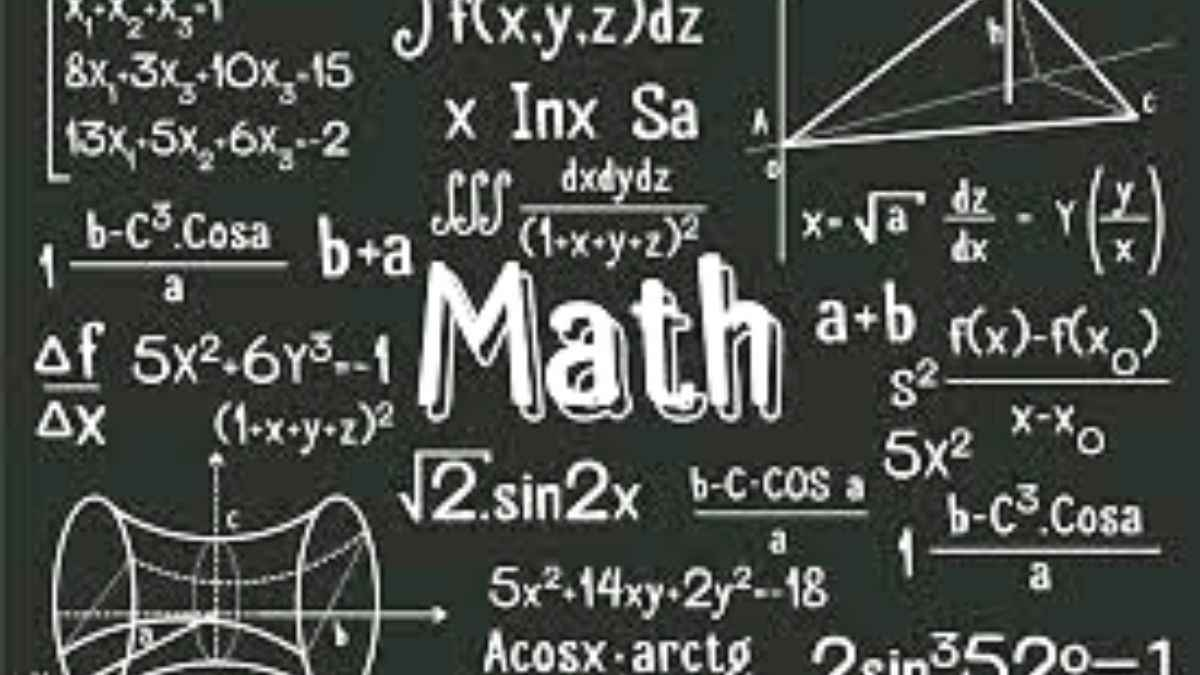# Math riddles with answers: These math riddles will compel you to rejoin your school math camp!

Do you recall those summer school camps where they used to teach you exciting math tricks and techniques? Meditate for 1 hour or time travel; simply bring back those tricks as those will come to use in these math riddles.Do you recall those days when back in school, you were perhaps very good or average at all other subjects? You were good at language, liked social studies, and had a thing for science, but the moment you looked at your math textbook, you would start sweating.

Whether you liked math in school or not, the subject has always demanded extra efforts, extra dedication, and extra hours of sleepless problem solving.

Math is a subject that comes with a wide array of concepts. If you wonder that you have understood one or two concepts in math, that would never imply that you will understand even a single word in the third one.

The subject demands both theoretical understanding and practical problem solving skills. Merely understanding the formulae or concepts is not what you need to score well; you are expected to solve even the toughest of math problem related to the same concept.

Have you ever envied those students who used to score excellent marks in math back in school? The hardest pill to swallow is when you find that the student who scores the highest marks in math is actually your best pal in school. What did you do in such a situation? Didn't you try hard to get well versed with the concepts in math?

Have you ever tried to get enrolled in summer clamps that help you improve your mathematical skills?

Well, we are sure a majority of you must have gone to such summer camps. What did you learn there? Were you taught abacus? Were you made to learn the math tables with the help of some easy and effective tricks? Or were you made to learn magic that always helps you land at the right answer?

Whatever your school camp made you learn, simply recall that, as the following math riddles will require you to make those tricks to use.

Yes, math is a subject that is not only tough but also interesting. However, some smart folks try to learn some math hacks, tricks, and techniques to solve tough math problems. Recall all those math tricks to solve these math riddles.

## Math riddles

Math Riddle 1: If you multiply this number by any other number, the answer will always be the same. What is the number?

Math Riddle 2: John has \$28.75. He purchased three cookies that cost \$1.50 each, five newspapers that each cost \$0.50, five flowers for \$1.25 each, and used the remainder of the cash on a pair of sunglasses. How much were the sunglasses?

Math Riddle 3: A monkey named Toto is trying to climb a coconut tree. He takes 3 steps forward and slips back 2 steps downward. Each forward step is 30 cm and each backward step is 40 cm. How many steps are required to climb a 100 cm tree?

Math Riddle 1: If you multiply this number by any other number, the answer will always be the same. What is the number?

Math Riddle 2: John has \$28.75. He purchased three cookies that cost \$1.50 each, five newspapers that cost \$0.50, five flowers for \$1.25 each, and used the remainder of the cash on a pair of sunglasses. How much were the sunglasses?

Math Riddle 3: A monkey named Toto is trying to climb a coconut tree. He takes 3 steps forward and slips back 2 steps downward. Each forward step is 30 cm and each backward step is 40 cm. How many steps are required to climb a 100 cm tree?